# KCSE 2010 Chemistry Paper 2 Questions with Marking Scheme

1. Which one of the following compounds; urea, ammonia, sugar and copper (II) chloride will conduct electric current when dissolved in water? Give a reason. (2 marks)
2. The diagram below shows an electrochemical cell. Study it and answer the questions that follow.Given the following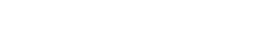1. Show on the diagram using an arrow, the direction flow of electrons. (1 mark)
2. Name two substances that are used to fill the part labeled L. (2 marks)

3. In an experiment to electroplate iron with silver, a current of 0.5 amperes was passed though a solution of silver nitrate for one hour.
1. Give two reasons why it is necessary to electroplate iron with silver. (2 marks)
2. Calculate the mass of silver that was deposited on iron (Ag = 108, 1 Faraday = 96,500 coulombs). (3 marks)

1. Give names for the following compounds:
1.(1 mark)

2.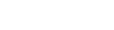(1 mark)

2. Describe a chemical test that can be carried out in order to distinguish between(2 marks)

3. Study the flow chart below and answer the questions that follow.1. Name the compounds: (2 marks)
1. L ………………………………………………..
2. N ……………………………………………….

2. Draw the structural formula of compound M showing two repeat units. (1 mark)
3. Give the reagent and the condition used in step 1. (1 mark)
4. State the reaction that takes place in: (2 marks)
1. Step 2;
2. Step 3.

4. The molecular compound of P is C2H2Cl4. Draw the two structural formulae of compound P. (2 marks)

1. Use the information in the table below to answer the questions that follow. The letters do not represent the actual symbols of the elements.1. Give reasons why melting points of:
1. S is higher than that of R; (1 mark)
2. V is lower than that of U. (2 marks)

2. How does the reactivity of W with chlorine compare with that of R with chlorine? Explain (2 marks)
3. Write an equation for the reaction between T and excess oxygen. (1 mark)
4. When 1.15g of R were reacted with water, 600cm3 of gas was produced.
Determine the relative atomic mass R. (Molar gas volume = 24 000 cm3) (3 marks)

5. Give one use of element V. (1 mark)

1. 50 cm3 of 1M copper (II) sulphate solution was placed in a 100cm3 plastic beaker. The temperature of the solution was measured. Excess metal A powder was added to the solution, the mixture was stirred, and the maximum temperature of the mixture measured. The experiment was repeated using powders of metals B and C. The results obtained are given in the table below: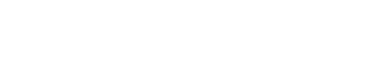1. Arrange the metals A, B, C and copper in order of the reactivity starting with the least reactive. Give reasons for the order. (3 marks)
2. Other than temperature change, state one other observation made when the most reactive metal was added to the copper (II) sulphate solution. (1 mark)

2. The standard enthalpy change of formation of methanol is -239kJmol-1.
1. Write the thermo chemical reaction for the standard enthalpy change of formation of methanol. (1 mark)
2. Methanol is manufactured by reacting carbon (II) oxide with hydrogen at 3000C and a pressure of 250 atmospheres.
The equation for the reaction is
CO(g) + 2H2(g) ----><---- CH3OH(g)

1. How would the yield of methanol be affected if the manufacturing process above is carried out at 3000C and a pressure of 400 atmospheres? Explain. (2 marks)
2. Use the following data to calculate the enthalpy change for the manufacture of methanol from carbon (II) oxide and hydrogen. (3 marks)3. The calculated enthalpy change in part b (ii) (II) above differs from the standard enthalpy change of formation of ethanol. Give a reason. (1 mark)

1. A student set up the apparatus as shown in the diagram below to prepare and collect dry ammonia gas.1. Identify two mistakes in the set up and give a reason to each mistake. (3 marks)
2. Name a suitable drying agent for ammonia. (1 mark)
3. Write an equation for the reaction that occurred when a mixture of ammonium chloride and calcium hydroxide was heated. (1 mark)
4. Describe one chemical test for ammonia gas. (1 mark)

2. Ammonia gas is used to manufacture nitric (V) acid, as shown below.1. This process requires the use of a catalyst. In which unit was the catalyst used? (1 mark)
2. Identify compounds A and B. (2 marks)
3. Using oxidation numbers, explain why the conversion of ammonia to nitric (V) acid is called catalytic oxidation of ammonia. (2 marks)
4. Ammonia and nitric (V) acid are used in the manufacture of ammonium nitrate fertilizer. Calculate the amount of nitric (V) acid required to manufacture 1000kg of ammonium nitrate using excess ammonia. (3 marks) (N = 14.0, H = 1.0, O = 16.0)

2. The melting and boiling points of zinc are 4190C and 9070C respectively. One of the ores of zinc is zinc blende. To extract zinc, the ore is fist roasted in air before feeding it into a furnace.
1. Write the formula of the main zinc compound in zinc blende. (1 mark)
2. Explain using an equation why it is necessary to roast the ore in air before introducing it into the furnace. (2 marks)

1. The diagram below shows a simplified furnace used in the extraction of zinc.
Study it and answer the questions that follow:1. Name two other substances that are also introduced into the furnace together with roasted ore. (1 mark)
2. The main reducing agent in the furnace is carbon (II) oxide. Write two equations to show how it is formed. (2 marks)
3. In what physical state is zinc at point Y in the furnace? Give a reason. (1 mark)
4. Suggest a value for the temperature at point X in the furnace. Give a reason. (1 mark)
5. State and explain one environmental effect that may arise from the extraction of zinc from zinc blende. (2 marks)
6. Give two industrial uses of zinc. (1 mark)

1. The diagram below shows how the rate of the following reactions vary with time.

A(g) + B(g) -----><------ 2C(g) + D(g)1. Which of the two curves represents the rate of the reverse reaction? Give a reason. (2 marks)
2. What is the significance of points X and Y on the figure? (2 marks)

2. State and explain the effect of an increase in pressure on the rates of the following reactions:
1. H2 (g) + Cl2 (g) -----> 2HCl (g)
2. CH3OH (l) + CH3COOH (l) -------> CH3COOCH3 (l)+ H2O (l)

3. In an experiment to study the rate of reaction between barium carbonate and dilute hydrochloric acid, 1.97g of barium carbonate were reacted with excess 2M hydrochloric acid. The equation for the reaction is
BaCO3(s) + 2HCl(aq) -----> BaCl2(aq) + CO2(g) + H2O(l)

The date in the table was obtained.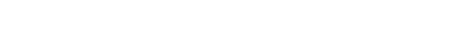1. On the grid provided, plot a graph of volume of gas produced (vertical axis) against time. (3 marks)2. From the graph determine the rate of reaction at:
1. 15 seconds; (1 mark)
2. 120 seconds (1 mark)
3. Give a reason for the difference between the two values. (1 mark)## MARKING SCHEME

1.
1. Ammonia (½) and Copper (II) Chloride (½)
This is because they form ions or ionise when they dissolve in water ( 1)
2.
1.2. Potassium nitrate solution, potassium chloride solution. Any soluble salt of potassium or sodium. Salt  (2 marks)
½ mark for mentioning the sa;lt without the taste.
3.
1.
• To improve its appearance/ make it attractive
• To prevent it from rusting / corrosion
2. Q = It
Mass = R.A.M x It
nF
= 108 x 0.5 x 60 x 60
1 x 96500
= 2.01g
2.
1.
1. 2, 2 - dimethyl propane/ dimethylpropane
2. pent - 2 - yne
2. Add acidified Potassium Manganate ( VII) or bromine to each of the compounds in seperate test tubes.Does not decolourise the reagents/ Purple colour remains/ reddish brown/yellow colour remains/ Burns with a blue / non- luminous flame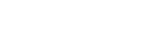Decolourises the reagents. Burns with a yellow/sooty/luminous flame
3.
1.
1.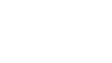Ethylethanoate
2.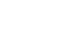Ethane
2.3. Water/steam and Conc. Sulphuric (VI) acid catalyst Phosphoric acid conditions. Heat, pressure 60-80, temperature 300°C
4.
1. Esterification/condensation
2. Substitution
4.3.
1.
1. Metallic bonds iin S are stronger than in R
2. V is monoatomic  (independent) hence weaker (1) van der waals forces while U is diatomic hence stronger van der waals forces V has less van der waals forces while U has more van der waal forces
2. W reacts more vigorously/ more reactive/ reacts faster
• Reactivity of group 1 elements increases down the group/ ionisation energy is less than that of R
• Easier to lose outer electron in W than in R. W is more electropositive than R
3. 4T(s) + 5O2(g) → 2T2O5(s)/T4O10(g)
4. 2R(s) = 2H2O(l) → 2ROH(aq) + H2(g)
Moles of gas =   600    = 0.025 Moles
24000
Moles of R = 2 × 0.025moles = 0.05 moles
R.A.M of R = 1.15 = 23
0.05
5. Used on flourescent tunbes/bulbs/lamps. Therefore used in arch welding/fire extingusher/preservatives in museums
4.
1.
1. B, A, Copper and C
• B has the highest ΔT
• C cannot displace the ions of Cu from solution there is no reaction
• A is more reactive than Cu because it displaces its ions from solution
2. Blue color of solution disappeared brown deposit is formed
2.
1. C(s) + 2H2(g) + ½O2(g) → CH3OH(g)ΔH = −239kJmol−1
2.
1. Yield increases/ will be higher therefore Equilibrium shifts tio the right/forwarrd reaction is formed
2. CO(g) + ½O2(g) → CO2 = −283kJmol−1
2H2 +O2(g) → 2H2O(l) = −572kJmol−1
CO2 + 2H2O(l) → CH3OH(l) + 3/2O2(l) = 715kJmol−1
Change in energy = 715 − 283 − 572 = −140kjmol−1
3. DHj of CO was not included
5.
1.
1.
• Water will condense into the hot flask and crack it
• Method of collection is wrong
• Ammonia is less dense than air
• Moist reactants should not be used
• The gas will be reabsorbed by water
2. CaO
3. 2NH4Cl(s) + Ca(OH)2(s) → 2NH3(g) + 2H2O(l) + CaCl2(g)
4. Pass dry HCl through ammonia/ take a glass rod/ pass it over a jar of ammonia and dip it in conc. HCl and white fumes are formed. Mixture forms white fumes
2.
1. Unit 1
2. A = NO
B = NO2
3. Nitrogen in (NH3) has oxidation
State of −3 while it has oxidation state of +5 in HNO3
Increase in oxidation state is oxidation
4. NH3(g) + HNO3(aq) → NH4NO3(aq)
Molar mass of NH4NO3 = 1000 × 1000
80
Moles of HNO3 = 1000 × 1000
80
Molar mass of HNO3  = 63
∴ mass of HNO3  = 1000 × 1000  ×  63
80
= 787.5kg

6.
1.
1. ZnS
2. So as to obtain ZnO which is easily reduced by CO to Zn
2ZnS(s) + 3O2(g) → 2Zn(s) + 2SO2(g)
2.
1.
• Coke/carbon
• Limestone/CaCO3
2. 2C(s) + O2(g) → 2CO(g)
CO2(g) + C(s) → 2CO(g)
3. Vapour/gas, temperature is above boiling point of Zinc
4. 600°C it is condensing/temperature is below boiling point of Zinc
5. Formation of gullies due to scooping of soil containing the ore/ CO2 leading to global warming
6.
• Making brass
• Making −ve terminal in dry cells
• Galvanization of iron sheets
7.
1. Curve I
1. The concentration of products are increasing
The rate of rxn is increasing
2. At time x equilibrium has been established, the rate of forward reaction is equal to the rate of reverse reaction and this has a value of Y.
2.
1.
• Increasing pressure increases rate of reaction.
• Molecules are brought closer, more collision of gases particles.
2. Incraesing pressure has no effect on liquids
3.Graph
1.
• Labelling axes
• Showing points correctly
• Smooth curve through the points
2.
1. at 15s, ( tangent drawn and used correctly)
2. at 120s, (tangent drawn and used correctly)
3. amount of BaCo3 is decreasing with time at 15s the value is higher than at 120s.

• ✔ To read offline at any time.
• ✔ To Print at your convenience
• ✔ Share Easily with Friends / Students